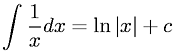Equations > Calculus > Integration/Integrals > Integral of reciprocal

### Integral of reciprocalLatex Code:

MathML Code:

 $\int \frac1x\mathrm{dx}=\mathrm{ln}|x|+c$

MathType 5.0: